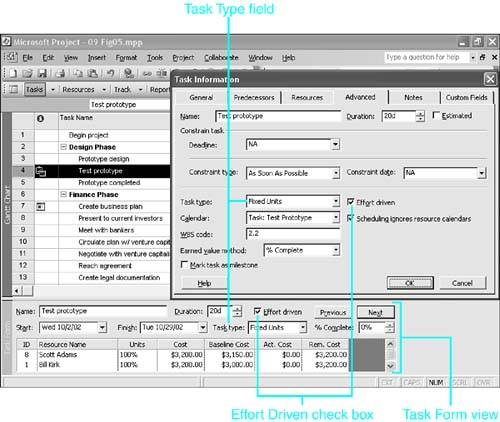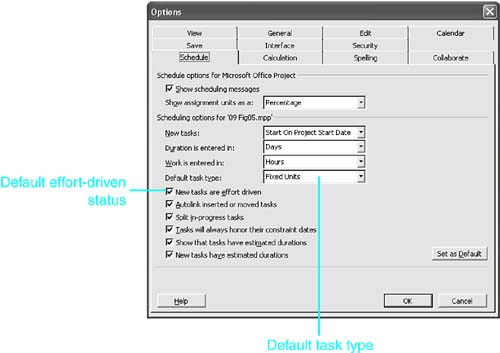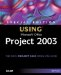<  Day Day Up  >

All tasks are one of three types, as defined in the Task Type field: Fixed Units (the default), Fixed Work, or Fixed Duration. Sometimes the task by its very nature falls easily into one of the three categories. Perhaps the task duration is set by a client, by the government, or by another organization, and you must work within that time frame. You could define that task as a fixed-duration task to keep Project from changing the duration. On the other hand, you might contract to provide a fixed amount of work for a client. In that case, you could define that task as a Fixed Work task so Project can't change the amount of work that is scheduled.

You can also use temporary changes in the Task Type field to control how Project responds to an assignment change. For instance, if you want to increase the duration for a task but keep the total amount of work constant, you can make it a Fixed Work task and then change the duration.

Table 9.1 summarizes which variable Project recalculates when you change a value in an existing assignment. The table assumes that your initial change is an increase in the respective variables (as indicated by the plus signs in that column). If your initial change is a decrease, you can simply reverse the plus and minus signs in the columns to the right.

The first column lists the variable that you might change. The three columns to the right show which variable Project recalculates as a result; the plus or minus sign indicates the direction of the resulting change. The formulas in Table 9.1 use an underline for the fixed variable, to help you follow the logic of the changes. Thus, in the first row, an increase in Duration for a Fixed Units task leads to an increase in Work, but for a Fixed Work task it leads to a decrease in Units.

##### Table 9.1. Task Types and Work Formula Calculations

Initial Change

Fixed Units Result

Fixed Work Result

Fixed Duration Result

+ Duration

+ Work

- Units

- Units

+ Units

- Duration

- Duration

+ Work

+ Work

+ Duration

+ Duration

+ Units

Formulas:

D x U = W

D x U = W

D x U = W

When an initial change is the fixed variable itself, the rule is a little more complicated. Those results are underlined in the table. Remember that duration is a task variable and that units and work are assignment variables. When you change one of the assignment variables (Units or Work), and it is the fixed variable, Project leaves the other assignment variable alone and changes task duration. When Duration is fixed and you change it, Project leaves the assignment work unchanged and adjusts the units.

As an example of how you can use this powerful tool, even in initial assignments, suppose you want to force Project to calculate the number of resource units you need to assign when you know the duration of the task and the amount of work you want done. You can make the task a Fixed Duration task and enter the amount of work. Project has to calculate the necessary units in order to keep the work formula in balance.

##### Figure 9.5. The task type can be set on the Advanced tab in the Task Information dialog box or in the Task Form view.As mentioned previously, the default task type for Project is Fixed Units, which means that new tasks are automatically assigned the Fixed Units task type. You can change the default type for new tasks in a project by selecting Tools, Options. In the Options dialog box, select the Schedule tab and the type you want as the default in the Default Task Type field (see Figure 9.6).

##### Figure 9.6. You can change the default task type in the Options dialog box.It might be helpful now to add recognition of task types to the rules about how the work formula is calculated. Project responds in the following ways when you create a new assignment for a task:

• If you enter a value in Units and leave the Work field blank, Project calculates the unknown Work (because both Units and Duration have been given values). This is true no matter what the task type.

• If you enter a value in Work and leave the Units blank, Project's calculation depends on the task type:

• If the task is not a Fixed Duration task (that is, if it's Fixed Units or Fixed Work), Project adjusts the Duration value. It assumes that the Units value is the default (100%, or less if the Max Units value is less) and calculates the Duration value that is necessary for the resource to complete the amount of work you assigned.

• However, if the task is a Fixed Duration task, Project cannot change the Duration entry, and it calculates the Units needed to complete the Work value you entered and places that value in the Units field.

• If you entered values in both Units and Work, Project again bases its calculations on the task type:

• If the task is not a Fixed Duration task, Project adjusts the Duration to accommodate the Work and Units values you entered.

• If the task is a Fixed Duration task, Project keeps the Work amount you entered and calculates a new Units value that can do the specified work in the given (fixed) duration.

 <  Day Day Up  >Special Edition Using Microsoft Office Project 2003
ISBN: 0789730723
EAN: 2147483647
Year: 2004
Pages: 283
Authors: Tim Pyron

Similar book on Amazon